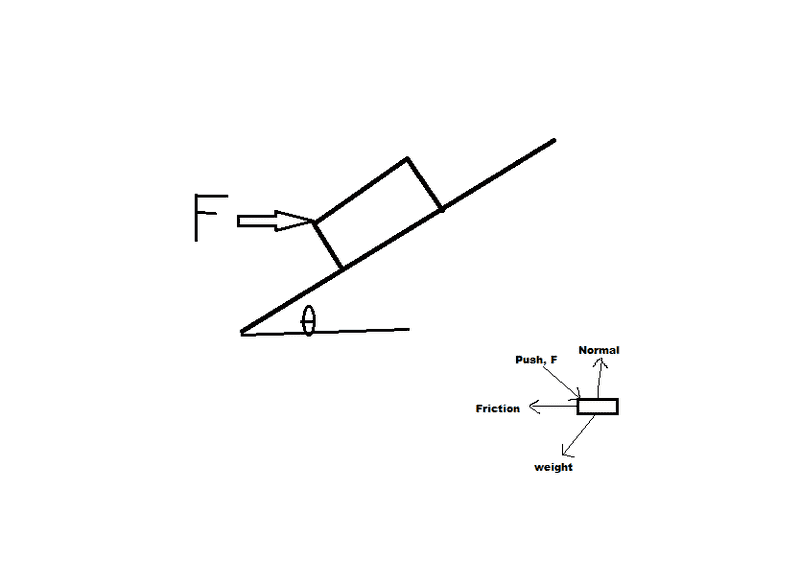# Pushing a crate up incline

• Nikstykal
In summary, A crate of mass m is pushed horizontally with force F up an inclined plane of θ from the ground. The plane has a friction coefficient of μ. To determine the normal force, N, acting on the crate, the free body diagram is set up with the normal force as the y-axis. This allows the equation ΣFy = may to be simplified to ay = 0, resulting in N = Fsinθ + mgcosθ. The acceleration, a, is then determined by substituting N into the equation ΣFx = max = -μ(Fsinθ + mgcosθ) + Fcosθ - mgsinθ.

## Homework Statement

Crate of mass m is pushed horizontally with force F up an inclined plane of θ from ground. The plane has a friction coefficient of μ.

a) draw free body diagram of crate
b) derive expression for normal force
c) derive expression for acceleration

*** Use ONLY m, g, F, μ, θ ****

ΣF = ma
|a| = √(ax2+ay2)

## The Attempt at a Solution[/B]
Included is a picture of the problem with my free body diagram.

I set the normal as the y-axis so that when solving ΣFy=may I can set ay=0.

ΣFx=max=-μN+Fcosθ-mgsinθ
ΣFy=may=N-Fsinθ-mgcosθ

ay=0 so N = Fsinθ+mgcosθ (part b)

Since ay=0, a=√ax2 = ax. Substituting N into ax:
max=-μ(Fsinθ+mgcosθ)+Fcosθ-mgsinθ

is this correct?

Looks correct.

Looks correct to me as well although I would have drawn the fbd at the same angle. Nothing wrong with yours it just look odd.

CWatters said:
Looks correct to me as well although I would have drawn the fbd at the same angle. Nothing wrong with yours it just look odd.
If you do that then you will have two components of acceleration (in the x AND y direction) and so your definition of N would involve more variables than the ones they allow, or at least would involve a lot more manipulation to isolate N.

Nikstykal said:
If you do that then you will have two components of acceleration (in the x AND y direction) and so your definition of N would involve more variables than the ones they allow, or at least would involve a lot more manipulation to isolate N.
mfb was only commenting on the orientation of the FBD. You can draw it with the same orientation as the physical arrangement but still choose the X and Y axes as parallel and normal to the plane.